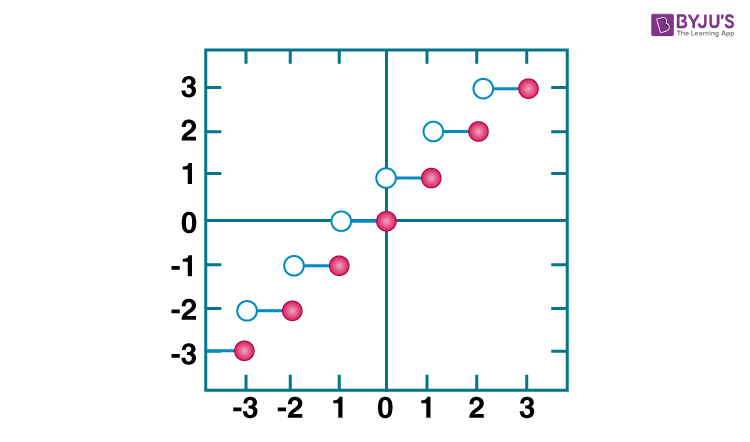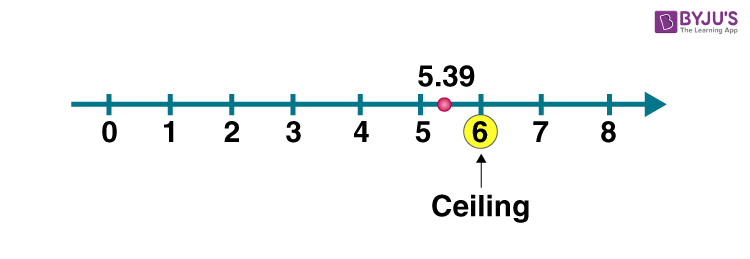# Ceiling Function

In Mathematics and Computer Programming, two important functions are used quite often. One is the floor function, and the other is the ceiling function. For example, the floor and ceiling of a decimal 3.31 are 3 and 4 respectively.  So with the help of these two functions, we get the nearest integer in a number line of a given decimal. In this article, let us discuss the ceiling function definition, notation, properties, graphs, and examples.

## Ceiling Function Definition

Ceiling function is a function in which the smallest successive integer is returned. In other words, the ceiling function of a real number x is the least integer that is greater than or equal to the given number x. The ceiling function is defined as:

f (x) = minimum { a ∈ Z ; a ≥ x }

### Ceiling Function Symbol

The ceiling function is also termed as the smallest integer function. The notation to represent this function is ⌈ ⌉. It can be used as ⌈x⌉ or ceil (x) or f(x) = ⌈x⌉

The symbol of floor function is also a type of square bracket. i.e.,  ⌊ ⌋.

## Ceiling Function Properties

Let us consider that x and y are two real numbers and ceil (x) = ⌈x⌉. Some of the important properties of the ceiling functions are:

• ⌈x⌉ + ⌈y⌉ – 1 ≤ ⌈x + y⌉ ≤ ⌈x⌉ + ⌈y⌉
• ⌈x + a⌉ = ⌈x⌉ + a
• ⌈x⌉ = a; iff x ≤ a < x + 1
• ⌈x⌉ = a; iff x – 1 < a ≤ x
• a < ⌈x⌉ iff a < x
• a ≤ ⌈x⌉ iff x < a

## Ceiling Function Formula

The formula to find the ceiling value for any given specified value is:

f (x) = minimum { a ∈ Z ; a ≥ x }

It is represented by:

f(x) = ⌈x⌉ = Least Nearest successive Integer of specified value

Example: Find the ceiling value of 3.7.

Given, x = 3.7

As we can see, the integer more than 3.7, are 4,5,6,7,..and so on.

The nearest integer is here 4.

Therefore, f(3.7) = ⌈3.7⌉ = 4

### Floor Function Formula

Floor function is the reverse function of ceiling function. It gives the largest nearest integer of the specified value.

It is represented by:

f(x) = ⌊x⌋ = Largest Nearest Integer of specified value

Example: Find the floor value of 3.7.

If we see, the number of integers less than 3.7, is 3,2,1,0 and so on.

So, the highest integer will be 3.

f(3.7) = ⌊3.7⌋ = 3

## Ceiling Function Graph

The graph of ceiling function is a discrete graph which consists of discontinuous line segments with one end with a dark dot (closed interval) and another end with an open circle (open interval). The ceiling function is a kind of step function since it looks like a staircase.

The graph of ceiling function is illustrated below:### Difference Between the Floor Function and the Ceiling Function

The ceil function and the floor function have a different definition.

• The ceil function returns the smallest value, whereas the floor function returns the largest value for the specified number. But the floor and ceiling of an integer remain the same. Suppose the floor and ceiling of 4 are 4 for both of them.
• Both the functions are represented by square brackets symbol, but with top and bottom parts missing.
• Also, another difference can be found using the graph. In the graph of ceiling function, it has an open dot on the left and the solid dot on the right. But for the floor function, it is vice versa. It means that the solid dot on the left and the open dot on the right.
• If 5.6 is a specified value, then the floor value is 5, and the ceiling value is 6.

### Solved Examples

Example 1:

Evaluate the ceiling function of 4.5 and – 4.5. Also, explain the answer.

Solution :

⌈4.5⌉ = 5 and ⌈- 4.5⌉ = – 4

Explanation:

The ceiling function of a real number is the least integer number greater than or equal to the given number.

In the case of 4.5, the integers greater than 4.5 are 5, 6, 7, 8, …..

The smallest of all is 5.

Therefore, ⌈4.5⌉ = 5.

In the case of -4.5, the integers that are greater than – 4.5 are – 4, – 3, – 2,…

The smallest of them is – 4

Therefore, ⌈- 4.5⌉ = – 4

Example 2: Explain the ceiling function of 5.39 with the help of the graph.

Solution:

⌈5.39⌉ = 6

It can be demonstrated on the number line as below:## Frequently Asked Questions – FAQs

### What does the ceiling function do?

The ceiling function gives the smallest nearest integer to the specified value in a number line. It gives the rounds up the given number.

### What does the floor function do?

The floor function also gives the greatest nearest value to the specified value in a number line which is above the specified value.

### How to calculate the ceiling value?

The ceiling function is related to the floor function by the formula:
⌈x⌉=−⌊−x⌋.

### What is the difference between ceiling function and floor function?

The ceiling function returns the smallest nearest integer whereas the floor function returns the largest nearest integer for a specified value.

### Give examples of floor and ceiling function.

If 2.6 is a specified value, then, ceiling value is equal to 3, and floor value is equal to 2.

Keep visiting BYJU’S – The Learning App and also download the app to get the interactive videos to learn with ease.